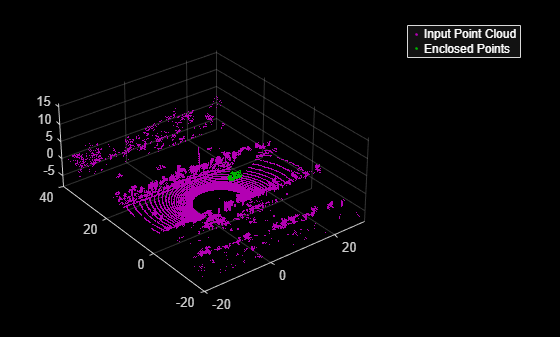# findPointsInsideCuboid

Find points enclosed by cuboid model

Since R2020b

## Syntax

``indices = findPointsInsideCuboid(model,ptCloudIn)``

## Description

example

````indices = findPointsInsideCuboid(model,ptCloudIn)` returns the linear indices of the points enclosed by a cuboid model, `model`, in an input point cloud, `ptCloudIn`.```

## Examples

collapse all

Extract points enclosed by a cuboid model in a point cloud. Create the cuboid model as a `cuboidModel` object.

Read point cloud data into the workspace.

`ptCloudIn = pcread('highwayScene.pcd');`

Define a cuboid model as a `cuboidModel` object.

```params = [11.4873085 8.59969 -1.613766 3.6712 1.3220... 1.75755, 0, 0, 0.017451]; model = cuboidModel(params);```

Find the points inside the cuboid.

`indices = findPointsInsideCuboid(model,ptCloudIn);`

Select the corresponding points in the input point cloud.

`cubPtCloud = select(ptCloudIn,indices);`

Plot the point cloud and the points enclosed by the cuboid.

```pcshowpair(ptCloudIn,cubPtCloud) xlim([-20 30]) ylim([-20 40]) legend("Input Point Cloud","Enclosed Points",'TextColor',[1 1 1])```## Input Arguments

collapse all

Cuboid model, specified as a `cuboidModel` object.

Point cloud, specified as a `pointCloud` object.

## Output Arguments

collapse all

Indices of enclosed points, returned as an N-element column vector. N is the number of enclosed points. Use the `select` function to select the corresponding points in the input point cloud `ptCloudIn`.

## Version History

Introduced in R2020b